# ISEE Upper Level Quantitative : How to find the diagonal of a cube

## Example Questions

### Example Question #1 : How To Find The Diagonal Of A Cube

What is the length of the diagonal of a cube with a side length of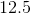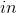? Round to the nearest hundreth.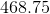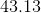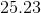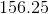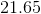Explanation:

It is easiest to think about diagonals like this by considering two points on a cube (if it were drawn in three dimensions).  We could draw it like this: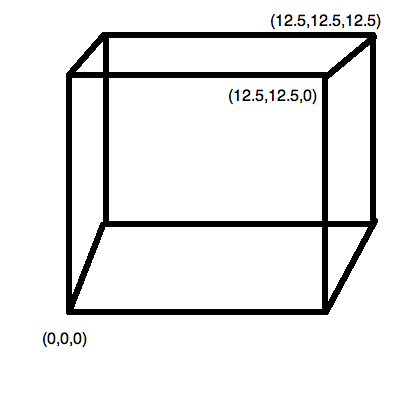(Note that not all points are drawn in on the cube.)

The two points we are looking at are: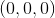and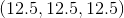Solve this by using the distance formula.  This is very easy since one point is alls.  It is merely: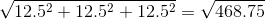This is approximately.

### Example Question #2 : How To Find The Diagonal Of A Cube

What is the length of the diagonal of a cube with a side length of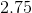? Round to the nearest hundreth.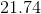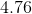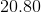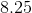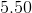Explanation:

It is easiest to think about diagonals like this by considering two points on a cube (if it were drawn in three dimensions). We could draw it like this: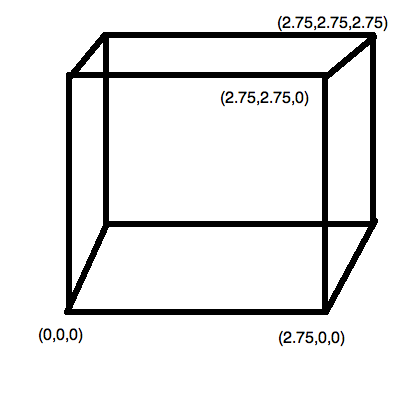(Note that not all points are drawn in on the cube.)

The two points we are looking at are:and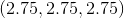Solve this by using the distance formula.  This is very easy since one point is alls.  It is merely: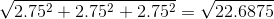This is approximately.

### Example Question #1 : How To Find The Diagonal Of A Cube

What is the length of the diagonal of a cube with a volume of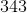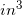? Round to the nearest hundredth.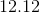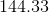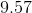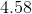Explanation:

First, you need to find the side length of this cube. We know that the volume is: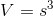, whereis the side length.

Therefore, based on our data, we can say: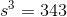Solving forby taking the cube-root of both sides, we get: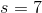Now, it is easiest to think about diagonals like this by considering two points on a cube (if it were drawn in three dimensions). We could draw it like this:

(Note that not all points are drawn in on the cube.)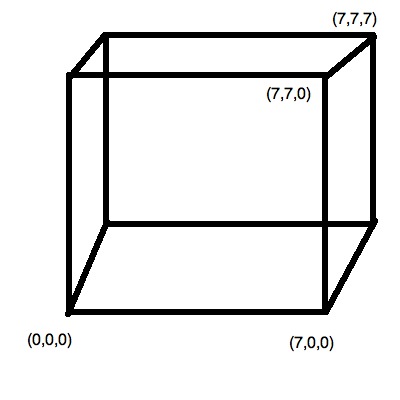The two points we are looking at are:and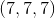Solve this by using the distance formula. This is very easy since one point is alls. It is merely: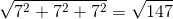This is approximately.

### Example Question #4 : How To Find The Diagonal Of A Cube

What is the length of the diagonal of a cube with a surface area of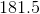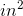? Round your answer to the nearest hundredth.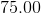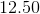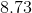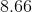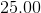Explanation:

First, you need to find the side length of this cube. We know that the surface area is defined by: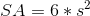, whereis the side length. (This is because the cube issides of equal area).

Therefore, based on our data, we can say: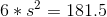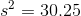Take the square root of both sides and get: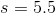Now, it is easiest to think about diagonals like this by considering two points on a cube (if it were drawn in three dimensions). We could draw it like this: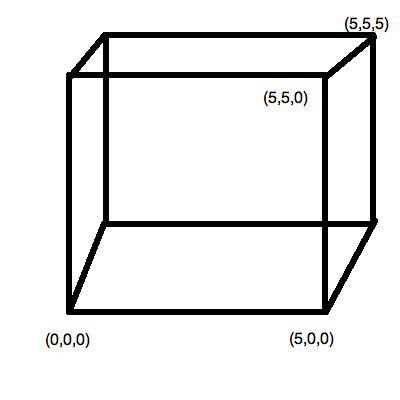(Note that not all points are drawn in on the cube.)

The two points we are looking at are:and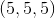Solve this by using the distance formula. This is very easy since one point is alls. It is merely: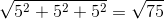This is approximately.